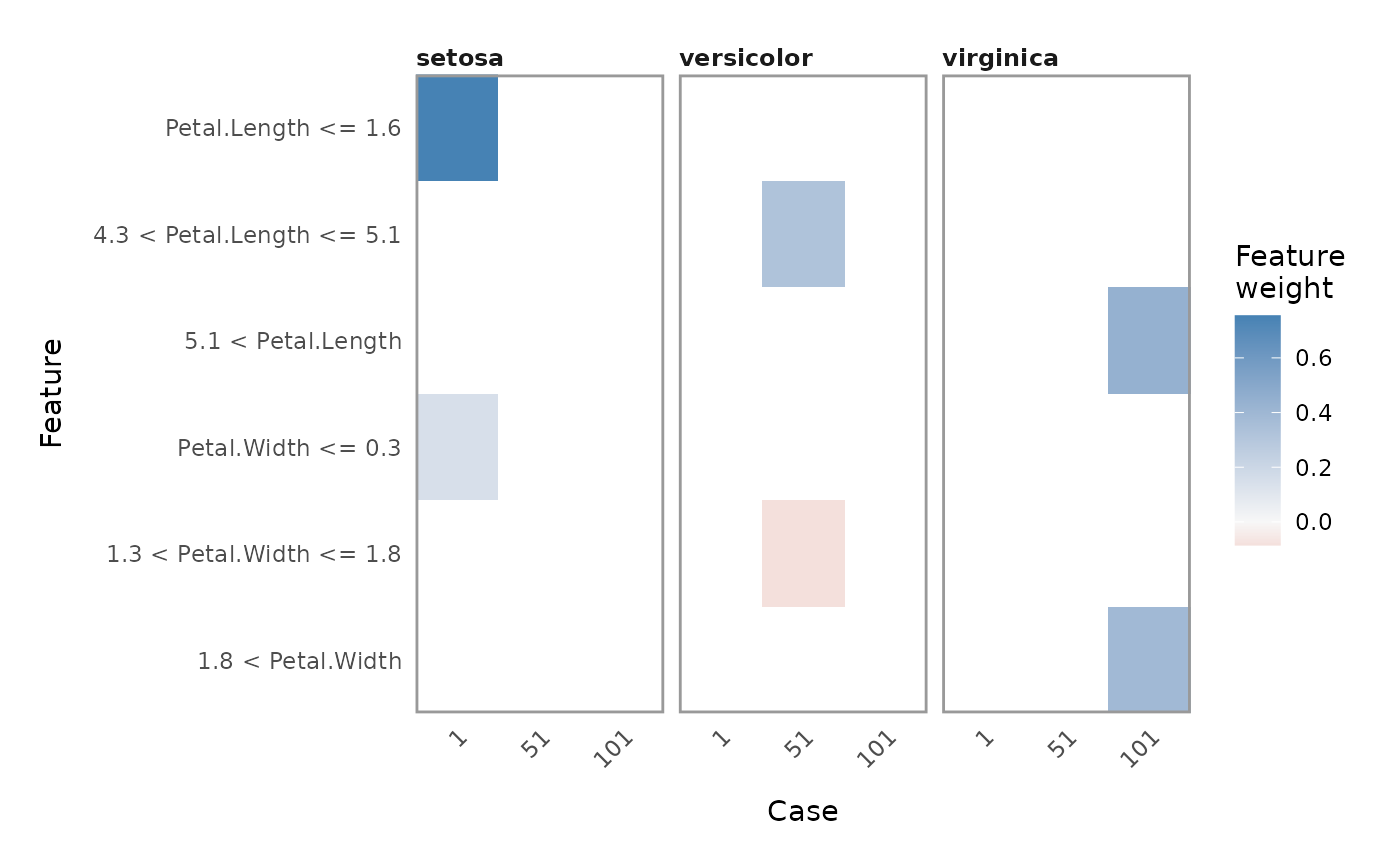This function produces a facetted heatmap visualisation of all case/label/feature combinations. Compared to plot_features() it is much more condensed, thus allowing for an overview of many explanations in one plot. On the other hand it is less useful for getting exact numerical statistics of the explanation.

plot_explanations(explanation, ...)

## Arguments

explanation A data.frame as returned by explain(). Parameters passed on to ggplot2::facet_wrap()

## Value

A ggplot object

Other explanation plots: plot_features(), plot_text_explanations()

## Examples

# Create some explanations
library(MASS)
iris_test <- iris[c(1, 51, 101), 1:4]
iris_train <- iris[-c(1, 51, 101), 1:4]
iris_lab <- iris[][-c(1, 51, 101)]
model <- lda(iris_train, iris_lab)
explanation <- lime(iris_train, model)
explanations <- explain(iris_test, explanation, n_labels = 1, n_features = 2)

# Get an overview with the standard plot
plot_explanations(explanations)